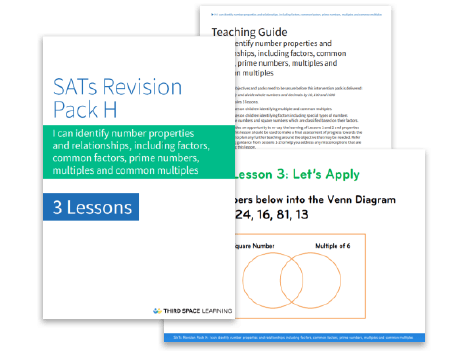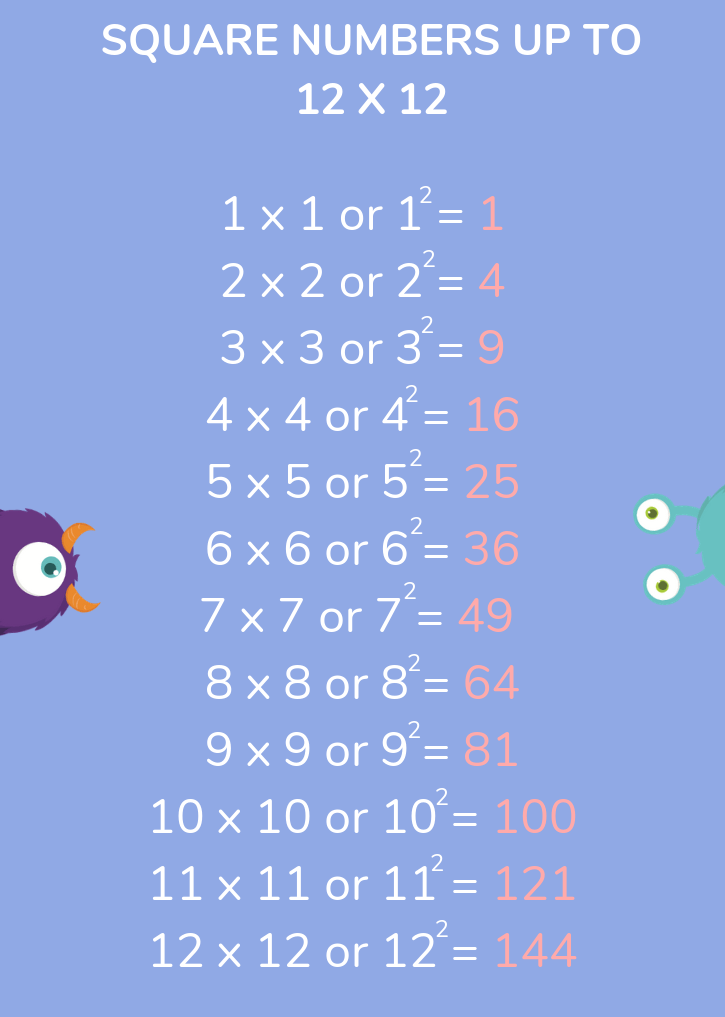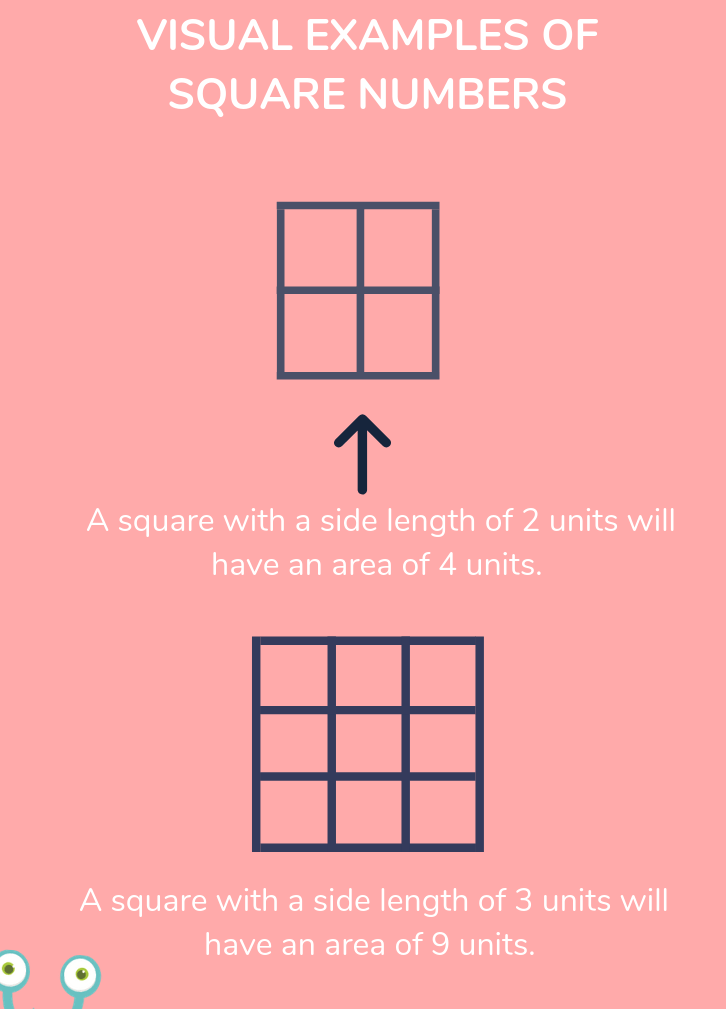Teaching support from the UK’s largest provider of in-school maths tuitionone to one lessonsschools supported

Consolidate learning and plug gaps

In-school online one to one maths tuition developed by maths teachers and pedagogy experts

See it in action

Hundreds of FREE online maths resources!

Daily activities, ready-to-go lesson slides, SATs revision packs, video CPD and more!Explore all resources
Four Operations

Consolidate learning and plug gaps

In-school online one to one maths tuition developed by maths teachers and pedagogy experts

See it in action

# What Is A Square Number? Explained For Primary School Parents And Kids

In this post we will be explaining what square numbers are, why they are called square numbers and providing all the information you’ll need to help your child get to grips with this area of maths. We’ve also included some square number based questions for your child to tackle, so take a look!

### What is a square number?

A square number is the result when a number has been multiplied by itself. For example, 25 is a square number because it’s 5 lots of 5, or 5 x 5. This is also written as 52 (“five squared”). 100 is also a square number because it’s 102 (10 x 10, or “ten squared”).

### Square number examples

5 examples of square numbers are 625 (25 x 25, 252), 90,000 (300 x 300, 3002), 289 (17 x 17, 172), 1 (1 x 1, 12) and 2304 (48 x 48, 482)FREE Factors, Multiples, Square & Cube Numbers Pack

Download this resource pack aimed at helping pupils identify number properties and relationships in advance of SATs. It includes teaching guidance, pupil practice sheets and activity slides.Download Free Now!

### Square numbers up to 12 x 12

The first 12 square numbers (and the ones children are most likely to know due to learning their times tables) are:

1 x 1 or 12 = 1
2 x 2 or 22 = 4
3 x 3 or 32 = 9
4 x 4 or 42 = 16
5 x 5 or 52 = 25
6 x 6 or 62 = 36
7 x 7 or 72 = 49
8 x 8 or 82 = 64
9 x 9 or 92 = 81
10 x 10 or 102 = 100
11 x 11 or 112 = 121
12 x 12 or 122 = 144

The square numbers from 1 to 100 are: 1, 4, 9, 25, 36, 49, 64, 81, 100

Here’s a handy image you can save with the above information on:### How do you square a number?

This is very simple. All you have to do is take the number, and multiply it by itself!

### Why are they called ‘square’ numbers?

What are ‘square’ numbers? Why are they not called (insert shape here) numbers?

These are questions that many primary school children ask, but fortunately the answer is simple. These particular collection of numbers are called square numbers (or squared numbers) for the simple reason that they form the area of a square. As squares have equal sides, calculating the area is simple – just “square” (multiply by itself) one of its sides!

For example, a square with side length 2cm would have an area of 4cm2 (as 22 = 4). In reverse, if we knew a square had an area of 9cm2, we’d know that each side would measure 3cm (as 32 = 9). See the diagrams below to demonstrate these examples.

You could use a 100 square to show this!### Square numbers for kids: When will my child learn about square numbers in primary school?

Whilst your child may learn about square numbers earlier, the National Curriculum doesn’t require schools to introduce square numbers until Year 5. As part of the multiplication and division topic, the curriculum states that pupils should be taught to:

• recognise and use square numbers and cube numbers, and the notation for squared (2) and cubed (3)
• solve problems involving multiplication and division including using their knowledge ofsquares and cubes

In the non-statutory notes and guidance, the curriculum advises that children understand the terms factor, multiple and prime, square and cube numbers and use them to construct equivalence statements (for example, 4 x 35 = 2 x 2 x 35; 3 x 270 = 3 x 3 x 9 x 10 = 92 x 10).

This knowledge will be built on in Year 6, particularly when learning about the order of operations, when children may learn the term ‘indices’ (an ‘index number’ is the name for the little 2 used to mean ‘squared’, or the little 3 used to mean ‘cubed’). By Year 5 and Year 6, children are expected to know not only the square numbers up to 12 x 12, but also the square numbers of multiples of 10 (20 x 20 = 400, 30 x 30 = 900 etc.)

### How do square numbers relate to other areas of maths?

Square numbers are particularly useful when finding the area of squares, which children begin to do in Year 4 (pupils should be taught to find the area of rectilinear shapes by counting squares) but which is built on further in Year 5 (pupils should be taught to calculate and compare the area of rectangles (including squares).

As previously mentioned, knowledge of square numbers is built on in Year 6 when pupils should be taught to use their knowledge of the order of operations (BODMAS) to carry out calculations.

Learning square numbers also gives children a firm base from which they can learn about cube or cubed numbers, but this will not usually happen until KS3.

Wondering about how to explain other key maths vocabulary to your children? Check out our Primary Maths Dictionary For Kids, or try these primary maths terms:

### Square number practice questions

1) 72 =

2) 36 and 64 are both square numbers. They have a sum of 100. Find two square numbers that have a sum of 130.

3) Here is a sorting diagram for numbers. Write a number less than 100 in each space.

4) Explain why 16 is a square number.

5) A question from the 2019 KS2 maths SATs paper testing children’s application of their knowledge of square numbers:

All of our resources can be found in the Third Space Maths Hub
Every teacher (and parent) at your school can create their own free Maths Hub account to access hundreds of free resources, guides and CPD videos. Premium Maths Hub access is available through a whole-school subscription or is included at no additional cost for schools using our 1-to-1 intervention programmes. Please note, if you are using Internet Explorer you will need to switch to another browser to use the Third Space Maths Hub. Enter your details here to register for free.

### Square number videos

Online 1-to-1 maths lessons trusted by schools and teachers
Every week Third Space Learning’s maths specialist tutors support thousands of primary school children with weekly online 1-to-1 lessons and maths interventions. Since 2013 we’ve helped over 150,000 children become more confident, able mathematicians. Learn more or request a personalised quote to speak to us about your needs and how we can help.

Primary school tuition targeted to the needs of each child and closely following the National Curriculum.##### Ellie Williams
Third Space Learning
Content Team
With a love for all things KS2 maths, Ellie is a part of the content team that helps all of the Third Space Learning blogs and resources reach teachers!

Related Articles

x#### FREE Factors, Multiples, Square & Cube Numbers Pack

Download this resource pack aimed at helping pupils identify number properties and relationships in advance of SATs. It includes teaching guidance, pupil practice sheets and activity slides.

#### FREE Factors, Multiples, Square & Cube Numbers PackDownload this resource pack aimed at helping pupils identify number properties and relationships in advance of SATs. It includes teaching guidance, pupil practice sheets and activity slides.